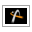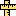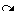AstroGrav Help /Menus /Window /UnitsVersion 5.0.1

# Window / Units   ()

Operating Systems: All
Window Types: All
Simulation Types: All

### Summary

Opens the units window of the frontmost simulation.

### Description

When you select this command, a window will be displayed showing the units of the frontmost simulation, and allowing you to change one or more of them. As you change the units, each of the frontmost simulation's open windows will be updated to reflect the change.

The units that can be edited and the data elements that they apply to are as follows.

1. Luminosity is a unit of power that applies to the following data elements.

2. Mass is a unit of mass that applies to the following data elements.

3. Radius is a unit of length that applies to the following data elements.

4. Position is a unit of length that applies to the following data elements.

5. Velocity is a unit of velocity that applies to the following data elements.

6. Period is a unit of time that applies to the following data elements.

7. Angle is a unit of angle that applies to the following data elements.
• Orbital Elements
• Orbital Inclination
• Asc Node Longitude
• Peri Longitude
• True Longitude
• Other Elements
• Eccentric Longitude
• Mean Longitude
• True Anomaly
• Eccentric Anomaly
• Mean Anomaly
• Peri Argument

8. Date is unit of time that applies to the current time as shown on the status bar in Solar System simulations, and Time is unit of time that applies to the current time as shown on the status bar in all other simulations.

• Edit / Undo()that undoes the latest change to the frontmost simulation.
• Edit / Redo()that redoes the most recently undone change to the frontmost simulation.